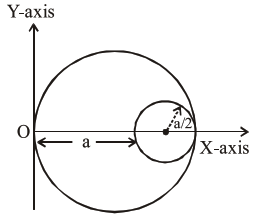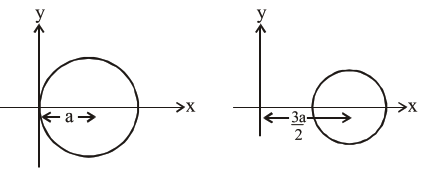# A circular hole of radius

Question:

A circular hole of radius $\left(\frac{a}{2}\right)$ is cut out of a circular disc of radius 'a' as shown in figure. The centroid of the remaining circular portion with respect to point ' $O^{\prime}$ will be :1. $\frac{1}{6} \mathrm{a}$

2. $\frac{10}{11} \mathrm{a}$

3. $\frac{5}{6} \mathrm{a}$

4. $\frac{2}{3} a$

Correct Option: , 3

Solution:Let $\sigma$ be the uniform mass density of disc then

$\mathrm{x}_{\mathrm{COM}}=\frac{\left(\sigma \pi \mathrm{a}^{2}\right) \mathrm{a}-\sigma \pi\left(\frac{\mathrm{a}^{2}}{4}\right) \times \frac{3 \mathrm{a}}{2}}{\sigma \pi \mathrm{a}^{2}-\frac{\sigma \pi \mathrm{a}^{2}}{4}}$

$=\frac{a-\frac{3 a}{8}}{1-\frac{1}{4}}=\frac{5 a}{6}$